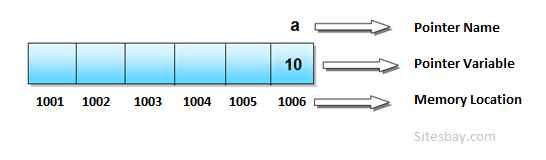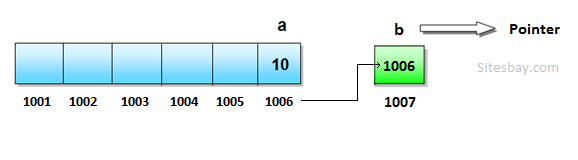# Pointer in C++

## Pointer in C++

### Pointer

To create linked list in C/C++ we must have a clear understanding about pointer. Now I will explain in brief what is pointer and how it works.A pointer is a variable that contains the address of a variable. Using pointer we can pass argument to the functions. Generally we pass them by value as a copy. So we cannot change them. But if we pass argument using pointer, we can modify them. To understand about pointers, we must know how computer store variable and its value. Now, I will show it here in a very simple way.

Let us imagine that a computer memory is a long array and every array location has a distinct memory location.

## Syntax

```int a = 10 // initialize variable a
```

It is like a house which has an address and this house has only one room. So address of house like this

• Name of the house: a
• Name of the person/value who live here is: 50
• House Number: 4010

If we want to change the person/value of this house, the conventional way is, type this code line

## Syntax

```a = 100 // new initialization
```

But using pointer we can directly go to the memory location of 'a' and change the person/value of this house without disturbing a. This is the main point about pointer. Use pointer like below code;

## Syntax

```int *b;    // declare pointer b
```

Here We transfer the memory location of a to b.

## Syntax

```b = &a; // the unary operator & gives the address of an object
```In above Images Integer pointer b store the address of the integer variable a. Now, we can change the value of a without accessing a.

## Syntax

```*b = 20;  // change the value of 'a' using pointer ‘b’
cout<
```

## Syntax

```#include <iostream.h>
using namespace std;

int main()
{
int a = 10;       // initialize integer variable a
cout<<"The value of 'a': "<
```

## Syntax

```The value of 'a': 10
The value of 'a' using *b: 20
```